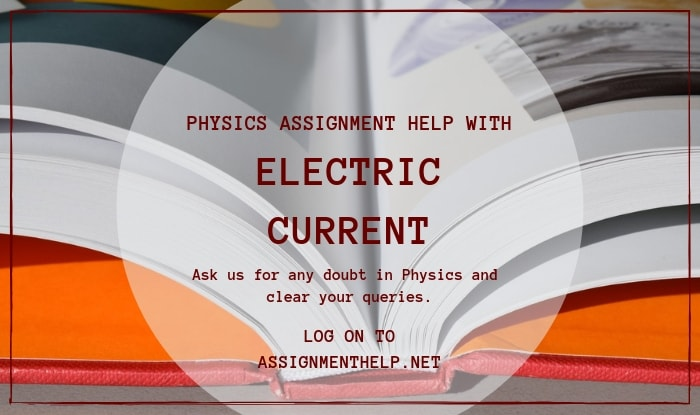# Physics Assignment Help With Electric Current

## 12.1 Electric Current

Charges in motion make an electric current. Time rate of flow of charge through a cross-section is called electric current.

Suppose, a charge Dq passes through a given cross-section area in a short time Dt, then the electric current is

I =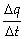More precisely, the instantaneous current at a given time t is

I =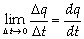### 12.1.1 Current Due to Translatory Motion of Charges

(i) If n particles, each having a charge q, pass through a given area in time t, the current is given by

I =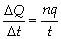(ii) If n particles, each having a charge q, pass per second per unit area, the current associated with cross-sectional area S is

I =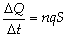### 12.1.2 Current Due to Rotatory Motion of Charge

If a point charge q is moving in a circle of radius r with speed v, then its time period T = (2pr/v).

So through a given cross-section (perpendicular to motion), the current is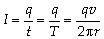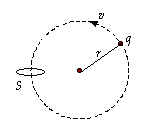### Email Based Assignment Help in Electric Current

We are the leading online Assignment Help provider. Find answers to all of your doubts regarding the Electric Current. Assignmenthelp.net provide homework, Assignment Help to the school, college or university level students. Our expert online tutors are available to help you in Electric Current. Our service is focused on: time delivery, superior quality, creativity and originality.